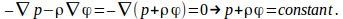## Total Force Acting on a Cube of Fluid

For a incompressible fluid at rest there are no shear or deforming forces and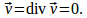Any stresses are normal to any surface drawn in the fluid and the pressure inside the fluid is the same in all directions.

Since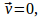though the fluid is incompressible the density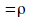need not be the same everywhere. We can writ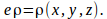Draw a cube in the fluid.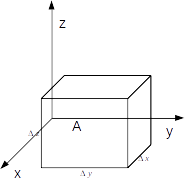The pressure on the face A is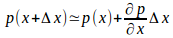and the force is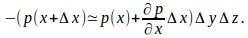The total force in the x direction is then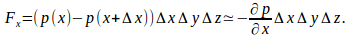Similarly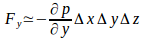and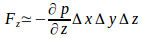The total force is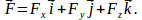As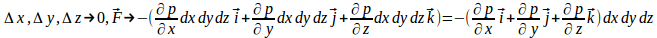Dividing by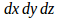gives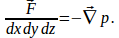This force must be balanced by an external force. If the external forces are due to a potential field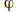the force per unit mass is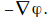The total force is then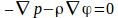since the fluid is in equilibrium.

If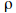is constant then